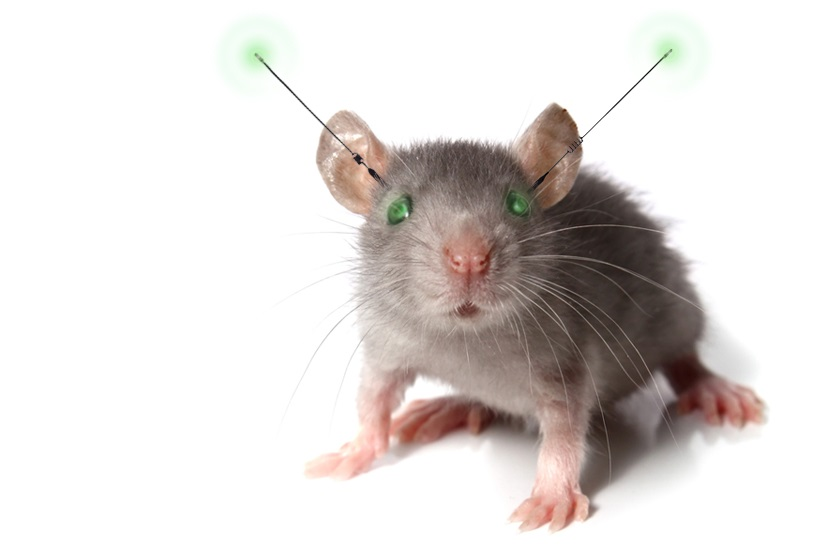## 18.7 Multiple Imputation using Chained Equations (MICE)### 18.7.1 Overview

• Generates multiple imputations for incomplete multivariate data by Gibbs sampling.
• Missing data can occur anywhere in the data.
• Impute an incomplete column by generating ‘plausible’ synthetic values given other columns in the data.
• For predictors that are incomplete themselves, the most recently generated imputations are used to complete the predictors prior to imputation of the target column.
• A separate univariate imputation model can be specified for each column.
• The default imputation method depends on the measurement level of the target column.

Your best reference guide to this section of the notes is the bookdown version of Flexible Imputation of Missing Data, by Stef van Buuren:

https://stefvanbuuren.name/fimd/ch-multivariate.html

For a more technical details about how the mice function works in R, see: https://www.jstatsoft.org/article/view/v045i03

### 18.7.2 Process / Algorithm

Consider a data matrix with 3 variables $$y_{1}$$, $$y_{2}$$, $$y_{3}$$, each with missing values. At iteration $$(\ell)$$:

1. Fit a model on $$y_{1}^{(\ell-1)}$$ using current values of $$y_{2}^{(\ell-1)}, y_{3}^{(\ell-1)}$$
2. Impute missing $$y_{1}$$, generating $$y_{1}^{(\ell)}$$
3. Fit a model on $$y_{2}^{(\ell-1)}$$ using current versions of $$y_{1}^{(\ell)}, y_{3}^{(\ell-1)}$$
4. Impute missing $$y_{2}$$, generating $$y_{2}^{(\ell)}$$
5. Fit a model on $$y_{3}$$ using current versions of $$y_{1}^{(\ell)}, y_{2}^{(\ell)}$$
6. Impute missing $$y_{3}$$, generating $$y_{3}^{(\ell)}$$
7. Start next cycle using updated values $$y_{1}^{(\ell)}, y_{2}^{(\ell)}, y_{3}^{(\ell)}$$

where $$(\ell)$$ cycles from 1 to $$L$$, before an imputed value is drawn.

### 18.7.3 Convergence

How many imputations ($$m$$) should we create and how many iterations ($$L$$) should I run between imputations?

• Original research from Rubin states that small amount of imputations ($$m=5$$) would be sufficient.
• Advances in computation have resulted in very efficient programs such as mice - so generating a larger number of imputations (say $$m=40$$) are more common Pan, 2016
• You want the number of iterations between draws to be long enough that the Gibbs sampler has converged.
• There is no test or direct method for determing convergence.
• Plot parameter against iteration number, one line per chain.
• These lines should be intertwined together, without showing trends.
• Convergence can be identified when the variance between lines is smaller (or at least no larger) than the variance within the lines.

### 18.7.4 Imputation Methods

Some built-in imputation methods in the mice package are:

• pmm: Predictive mean matching (any) DEFAULT FOR NUMERIC
• norm.predict: Linear regression, predicted values (numeric)
• mean: Unconditional mean imputation (numeric)
• logreg: Logistic regression (factor, 2 levels) DEFAULT
• logreg.boot: Logistic regression with bootstrap
• polyreg: Polytomous logistic regression (factor, >= 2 levels) DEFAULT
• lda: Linear discriminant analysis (factor, >= 2 categories)
• cart: Classification and regression trees (any)
• rf: Random forest imputations (any)